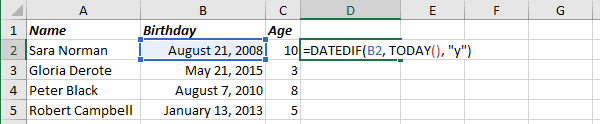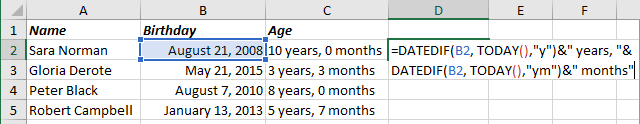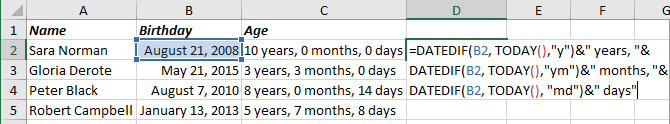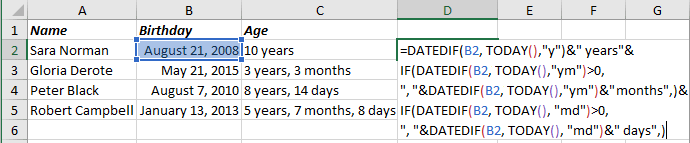# How to calculate age in Excel

Excel
To calculate the age of a person, a pet, a project, or even some device, you need to compute a number of complete years. The issue is that it doesn't seem enough to calculate a year's difference. Excel proposes a very useful formula that can help you to calculate that very fast.

The formula DATEDIF calculates days, months or years between two dates:

= DATEDIF (<start date>, <end date>, unit)

The parameter unit can be:

• "Y" - The number of full years in the period.

To calculate an age:

= DATEDIF (<start date>, TODAY (), "y")Note: The formula TODAY () returns the current date. Sure, you can enter the end date to calculate age, not for the current date.

• "YM" - The difference in months between <start date> and <end date> ignoring years. So, it looks like Excel changes a year for <start date> to the same as in <end date> and calculates the difference in days.

For example:

= DATEDIF (<start date>, TODAY (), "ym"):• "MD" - The difference in days between <start date> and <end date> ignoring months and years. So, it looks like Excel changes year and month for <start date> to the same as in <end date> and calculates the difference in days.

For example:

= DATEDIF (<start date>, TODAY (), "md"):Note: You can add IF to ignore months and days with 0:

= IF (<comparison>, <result if comparison is TRUE>, <result if comparison is FALSE>)